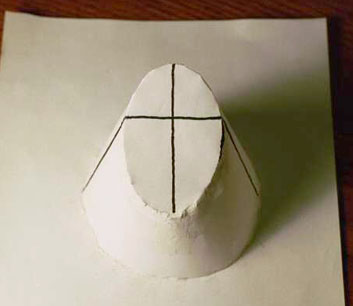Subject: Ellipse as a conic section I am 78 years old, +/- 10 years, depends how I feel! I have made a paper model of a cone, cut a sloping section, and removed the top. I have drawn the major and minor axis on the paper surface of the section. The major axis is not symmetrical about the minor axis. To me, this is not an ellipse. To me, an ellipse is a tubular section, because this gives a symmetrical major axis. What is your opinion? I am including an attachment of a picture I took of the model.Bruce Hi Bruce, I have two answers to your question, one from Walter and the other from Chris. There are two possibilities - which I cannot distinguish from the one image: (a) the minor axis is misindentified in the cross section; (b) the cross section is not 'flat' (or the cone is not truly circular). There is no doubt that a true plane cut of a circular cone has an equation which is quadratic: x2 + y2 = z2 is the cone. If Ax + By + Cz = D is the plane then x2 + y2 = (Ax+By -D)2 Simplified, this is a quadratic equation. If it is a finite closed curve it must be an ellipse. There are synthetic proofs of the fact that it is an ellipse as well. Walter Whiteley The ancient Greeks DEFINED a conic section to be the plane section of a cone. To relate the object you constructed to the more familiar approach to conics that is usually taught in school, you put two spheres inside your cone. One is tangent to the sides of the cone touching the plane from above, the other tangent below. the two points of tangency are the foci of your ellipse. One axis of the ellipse comes from the line joining those two foci; the other is the perpendicular bisector of that axis. Chris Go to Math Central